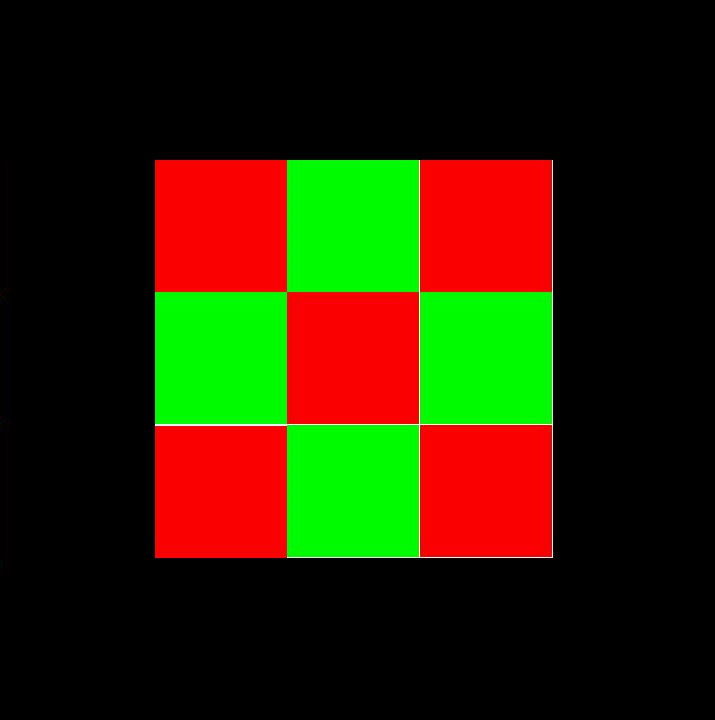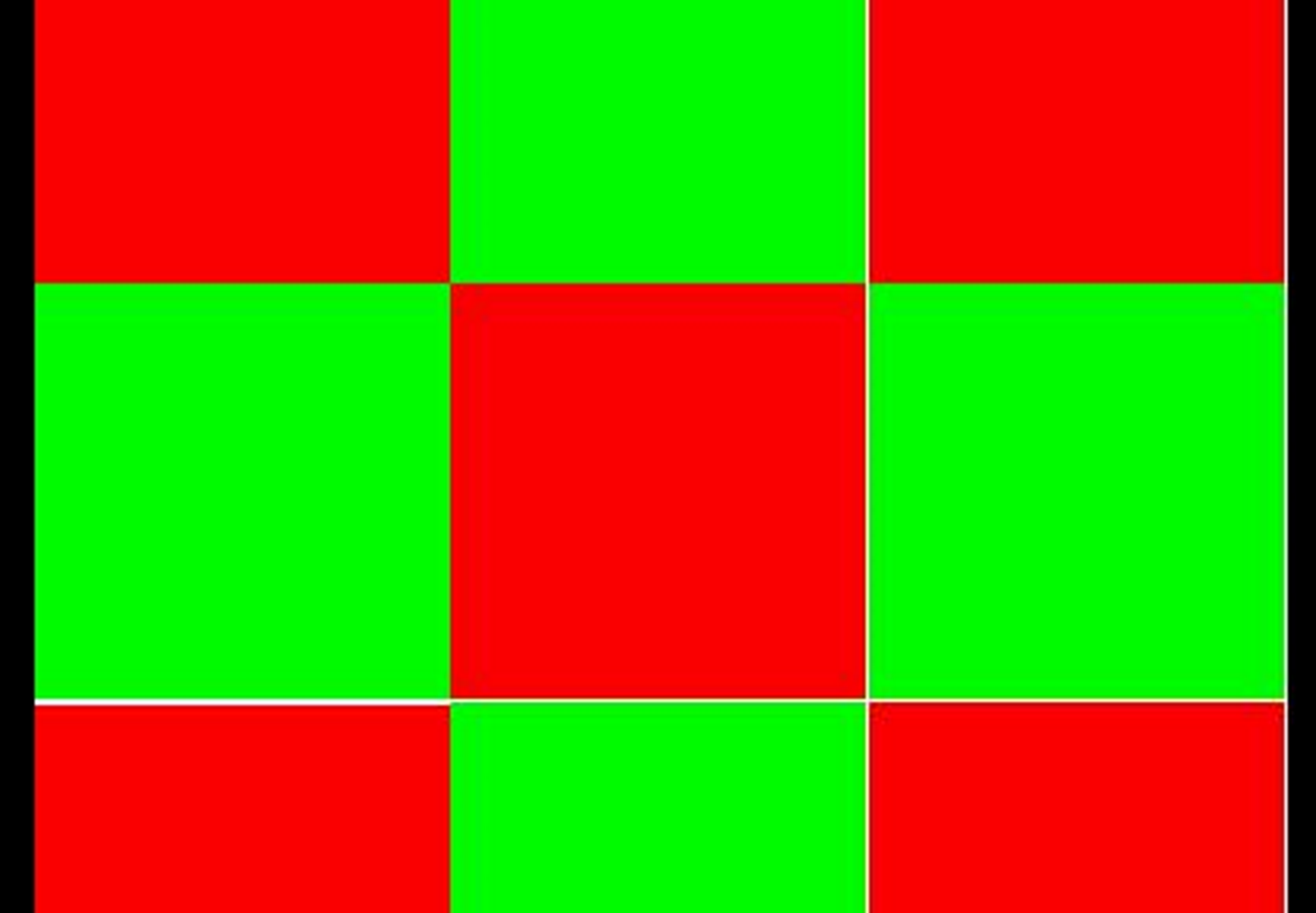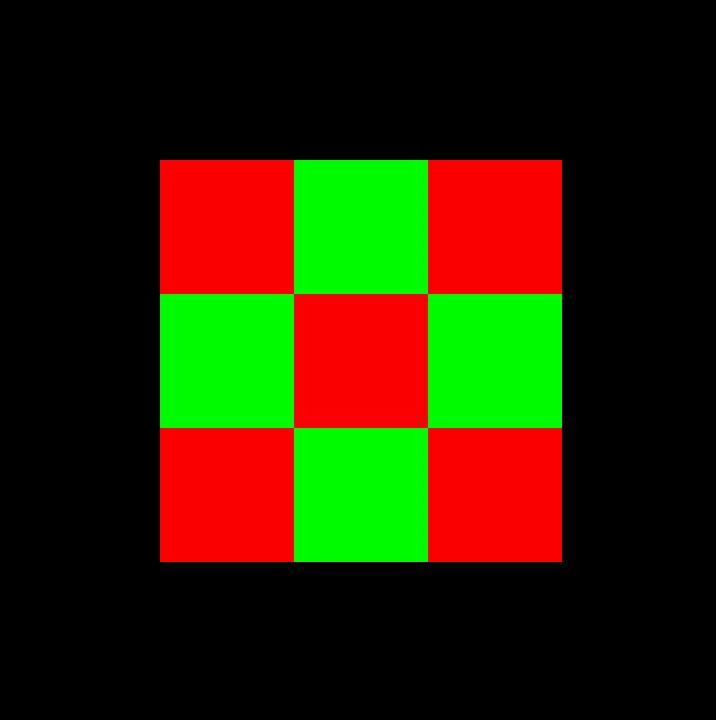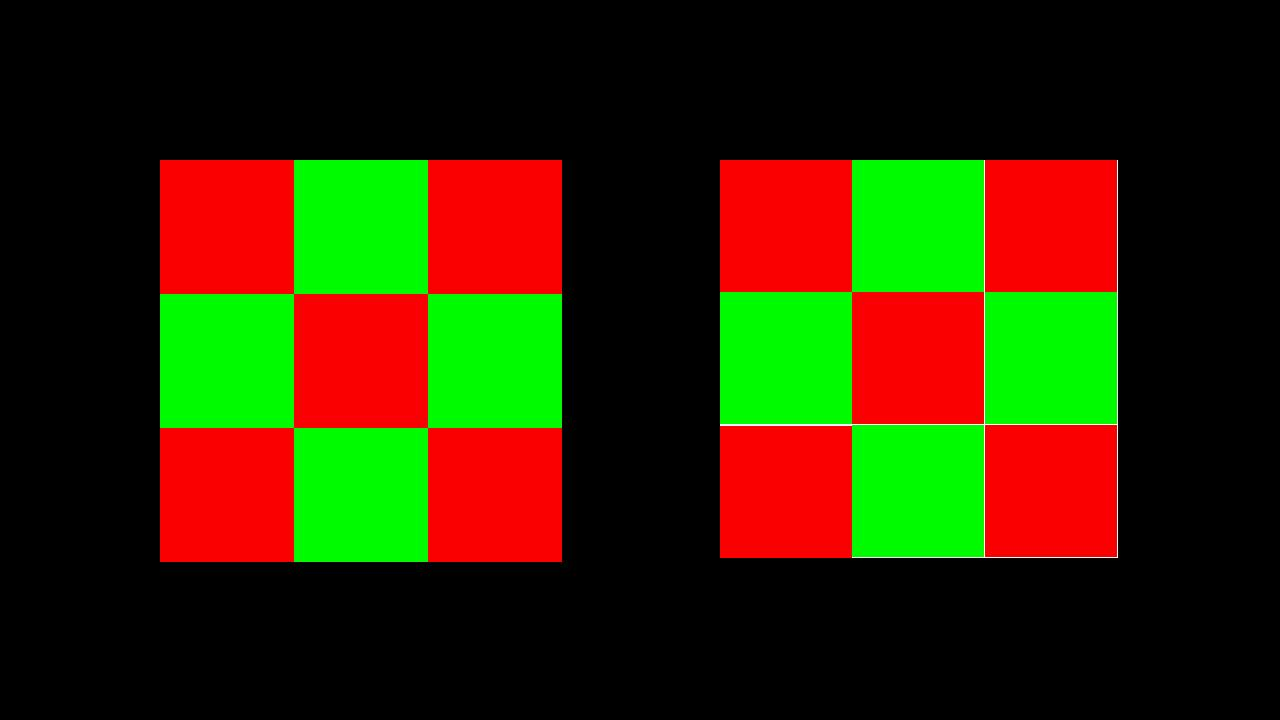# Roku: A Different Kind of 'Rule of Thirds'

Roku devices support a few different resolutions for displaying your app's UI:

• FHD (1920x1080)
• HD (1280x720)
• SD (720x480)

NOTE: This post is only going to focus on FHD and HD. Since SD is a different aspect ratio (4:3) and most Roku devices cannot output SD anyway, it is usually easier to omit explicit support and let the Roku autoscale the UI to handle SD.

Although you can provide explicit layouts for both HD and FHD, a much more common approach is to only provide the FHD layout and let the Roku downscale the UI to handle HD automatically. To do this, simply add this to your `manifest` file:

`ui_resolutions=FHD`

Now, you can simply use a 1920x1080 grid when specifying your UI dimensions and Roku will automatically handle converting it to HD as necessary. In order to autoscale the UI down, all of the dimensions are essentially multiplied by 2/3. So an element that is half-a-screen wide in and FHD app would be specified as 960 (1920/2), but would correctly render at 640 (960 * 2/3) on an HD screen.

However, there is a catch with this approach. Since everything is multiplied by 2/3, the original element dimensions must always be divisible by 3 to avoid rounding issues. Consider the following layout which renders a 3x3 grid - it is 597px wide (which is divisible by 3 since 5+9+7=12, which is divisible by 3) and thus each square is 199x199:

``````<Rectangle width="597" height="597" color="0xFFFFFF">
<Rectangle width="199" height="199" color="0xFF0000" translation="[0,0]"/>
<Rectangle width="199" height="199" color="0x00FF00" translation="[199,0]"/>
<Rectangle width="199" height="199" color="0xFF0000" translation="[398,0]"/>
<Rectangle width="199" height="199" color="0x00FF00" translation="[0,199]"/>
<Rectangle width="199" height="199" color="0xFF0000" translation="[199,199]"/>
<Rectangle width="199" height="199" color="0x00FF00" translation="[398,199]"/>
<Rectangle width="199" height="199" color="0xFF0000" translation="[0,399]"/>
<Rectangle width="199" height="199" color="0x00FF00" translation="[199,398]"/>
<Rectangle width="199" height="199" color="0xFF0000" translation="[398,398]"/>
</Rectangle>
``````If you look closely, you can see there are some white lines along the right edge of the grid. Zooming in on the image, we can see:The squares are not lining up correctly since 199 is not divisible by 3. Let's work through what is going on.

• The overall grid is 597x597 which is divisible by 3. The HD grid should end up 597 * 2/3 = 398px wide
• In order to make a 3x3 grid, each square is 199x199 - 199 is not divisible by 3
• 199 * 2/3 = 132.66666667 which gets rendered as 132
• The top left square ends up 132x132 at position 0,0
• The top middle square ends up rendered at position 132,0 so there is no gap
• The top right square should have been rendered at 398,0, which converts to 265 in HD. But since the first two squares were only 132 each, they only take up 264px so there ends up being a 1px gap between the second and third columns.
• Same thing happens between the second and third rows
• The top right square gets positioned at 265, but since it is only 132px wide, it only fills in to 397, leaving a 1px gap along the right edge (same along the bottom row)

So if we adjust our dimensions so that each individual square is divisible by 3 in both dimensions, we get:

``````<Rectangle width="603" height="603" color="0xFFFFFF">
<Rectangle width="201" height="201" color="0xFF0000" translation="[0,0]"/>
<Rectangle width="201" height="201" color="0x00FF00" translation="[201,0]"/>
<Rectangle width="201" height="201" color="0xFF0000" translation="[402,0]"/>
<Rectangle width="201" height="201" color="0x00FF00" translation="[0,201]"/>
<Rectangle width="201" height="201" color="0xFF0000" translation="[201,201]"/>
<Rectangle width="201" height="201" color="0x00FF00" translation="[402,201]"/>
<Rectangle width="201" height="201" color="0xFF0000" translation="[0,402]"/>
<Rectangle width="201" height="201" color="0x00FF00" translation="[201,402]"/>
<Rectangle width="201" height="201" color="0xFF0000" translation="[402,402]"/>
</Rectangle>
``````Here are the two grids side by side for comparison:Note that this applies to all UI dimensions, not just `width` and `height`. Any property that specifies a UI dimension is affected, including `translation` and `scale`. `scale` is particularly tricky because the resulting post-scaled dimension must be divisible by 3. So:

• 600 ✔️ with `scale` of `0.8` = 480 ✔️ * 2/3 = 320 ✔️
• 597 ✔️ with `scale` of `0.8` = 477.6 ❌